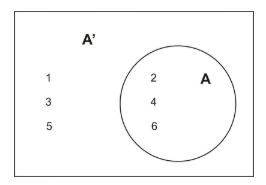#### Explain the complement of a set in simple words? It is really getting hard for me !!In set theory, the complement of A means, the elements which are not present in A. It is denoted by A'
For example-
Let say, A = {= natural number}
So, complement of A  = A' = {0}Here A = 2, 4, 6
And A' (complement of A) = elements which are not present in A  = 1, 3, 5